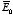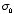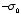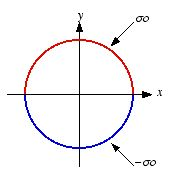# Home Work Set # 7, Physics 217, Due: October 31, 2001

### Problem 1

Find the potential outside an infinitely long metal pipe, of radius R, placed at right angles to an otherwise uniform electric field. Find the surface charge induced on the pipe.

### Problem 2

Find the potential outside a charged metal sphere (charge Q, radius R) placed in an otherwise uniform electric field. Explain clearly where you are setting the zero of the potential.

### Problem 3

A long cylindrical shell of radius R carries a uniform surface chargeon the upper half, and an opposite surface chargeon the lower half (see Figure 1). Find the electric potential inside and outside the cylinder.Figure 1. Problem 3.

### Problem 4

In Example 3.9 of Griffiths we derived the exact potential of a spherical shell of radius R which carries a surface charge.
a) Calculate the dipole moment of this charge distribution.
b) Find the approximate potential, at points far from the sphere, and compare this with the exact answer (eq. (3.87) of Griffiths). What can you conclude about the higher multipoles?

### Problem 5

A "pure" dipole p is situated at the origin, pointing in the z direction.
a) What is the force on a point charge q at (a, 0, 0) (Cartesian coordinates)?
b) What is the force on q at (0, 0, a)?
c) How much work does it take to move q from (a, 0, 0) to (0, 0, a)?